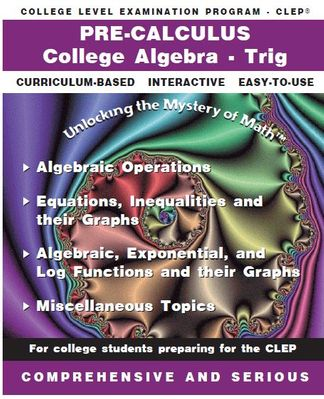Tutorial math and reading software for elementary and secondary arithmetic, basic math, algebra, geometry, precalculus plus GED, ABE, and CLEP preparation for elementary school, high school, college, adult education, and homeschool students.

# CLEP - PrecalculusIncludes Trig and College Algebra CLEP ProgramsItem# CLEP-PreCalc
Regular price: \$139.00
Limited Time Offer: \$69.50
Media type::

## Product Description

To satisfy the requirements and study the topics necessary for the precalculus CLEP exam, purchase this combination package of the CLEP College Algebra program and the Trigonometry program. The examination and this computer software covers material usually taught in a one-semester precalculus course that includes both college algebra and trig topics. Such a course is usually taken by students who have studied algebra and geometry in high school, but who need additional study of precalculus mathematics before enrolling in calculus and other advanced courses at the college level. The test and this software package contain the same topics as the CLEP precalculus exam. When you purchase this precalculus product, you recieve two software packages (College Algebra and Trig) at a bundle price break of \$139.
The College Board's College-Level Examination Program® (CLEP) introduced a new Precalculus Exam in January 2006. The new exam replaces the CLEP College Algebra-Trigonometry and Trigonometry exams. Why Should You Take CLEP Precalculus? Depending on your institution's policy: (1) Show your college math professors your passing score on the exam and allow you to opt out of a math proficiency core requirement (2) Prove your readiness for a Calculus class (3) Earn you three college credits

Description of the Examination
The Precalculus examination evaluates your mastery of skills and concepts required for success in a first-semester calculus course.

A large portion of the exam is devoted to testing your understanding of functions and their properties. Many of the questions test your knowledge of specific properties of the following types of functions: linear, quadratic, absolute value, square root, polynomial, rational, exponential, logarithmic, trigonometric, inverse trigonometric, and piecewise-defined. Questions on the exam will present these types of functions symbolically, graphically, verbally, or in tabular form. A solid understanding of these types of functions is at the core of all precalculus courses, and is a prerequisite for enrolling in calculus and other college-level mathematics courses.

The examination contains 48 questions, in two sections, to be answered in 90 minutes. Any time you spend on tutorials and providing personal information is in addition to the actual testing time.

Section 1: 25 Questions: 50 minutes. The use of an online graphing calculator is allowed for this section. Only some of the questions will require the use of the calculator.
Section 2: 23 Questions: 40 minutes. No calculator is allowed for this section. Although most of the questions on the exam are multiple-choice, there are some questions that require you to enter a numerical answer.

Knowledge and Skills Required

Questions on the examination require you to demonstrate the following abilities:

Recalling factual knowledge and/or performing routine mathematical manipulation

Solving problems that demonstrate comprehension of mathematical ideas and/or concepts Solving nonroutine problems or problems that require insight, ingenuity, or higher mental processes

The subject matter of the Precalculus examination is drawn from the following topics. The percentages indicate the approximate percentage of exam questions on that topic.

Approximate Percent of Examination

20% Algebraic Expressions, Equations, and Inequalities Ability to perform operations on algebraic expressions, equations, and Inequalities Ability to solve equations and inequalities, including algebraic expressions linear, quadratic, absolute value, polynomial, rational, radical, exponential Ability to solve systems of equations, logarithmic, and trigonometric including linear and nonlinear

15% Functions: Concept, Properties, and Operations Ability to demonstrate an understanding of the concept of a functionOperations function, the general properties of functions (e.g., domain, range), function notation, and to perform symbolic operations with functions (e.g., evaluation, inverse functions)

30% Representations of Functions: Symbolic, Graphical Ability to recognize and perform operations and transformations
Functions are presented symbolically, graphically, or in tabular form to demonstrate an understanding of basic properties of functions and to recognize elementary functions (linear, quadratic, absolute value, square root, polynomial, rational, exponential, logarithmic, trigonometric, inverse trigonometric, and piecewise-defined functions) that are presented symbolically

10% Analytic Geometry an understanding of the analytic geometry of lines, circles, parabolas, Ability to analyze and graph ellipses, and hyperbolas

15% Trigonometry and its Applications Demonstrate an understanding of the basic trigonometric functions and their inverses and to apply the basic trigonometric ratios and identities Ability to apply trigonometry in various right triangles and on the unit circle Ability to interpret using trigonometry in problem-solving contexts

10% Functions as Models construct functions as models and to translate ideas among symbolic, graphical, tabular, and verbal representations of functions
FAQ
Typically, how long does it take to complete this particular computer software program? Will this software program prepare me for the precalculus clep test?